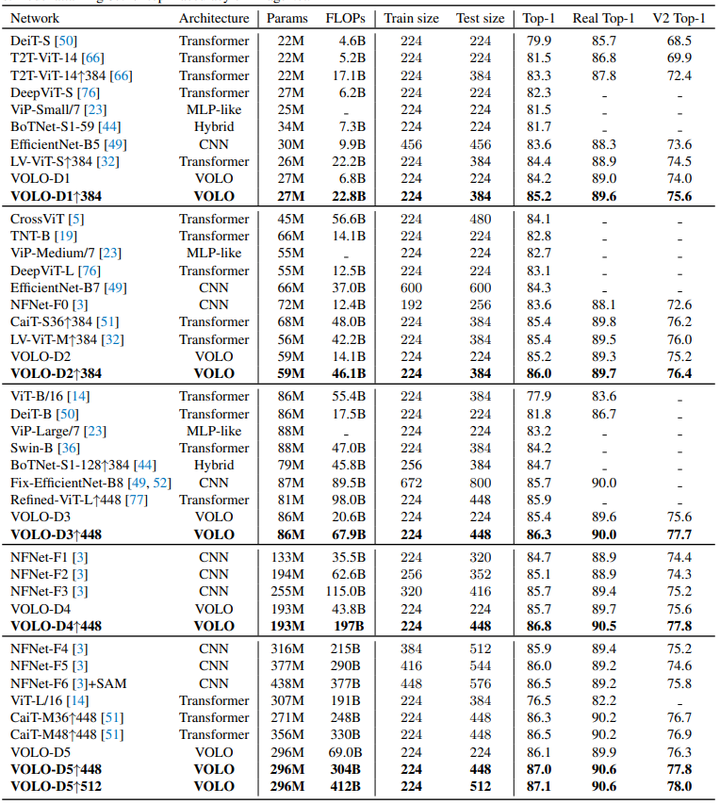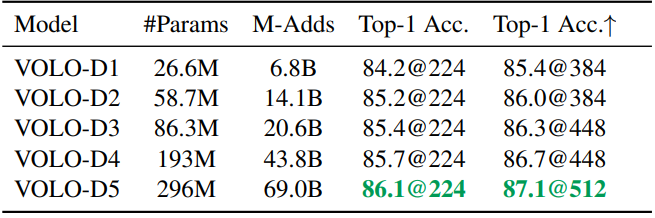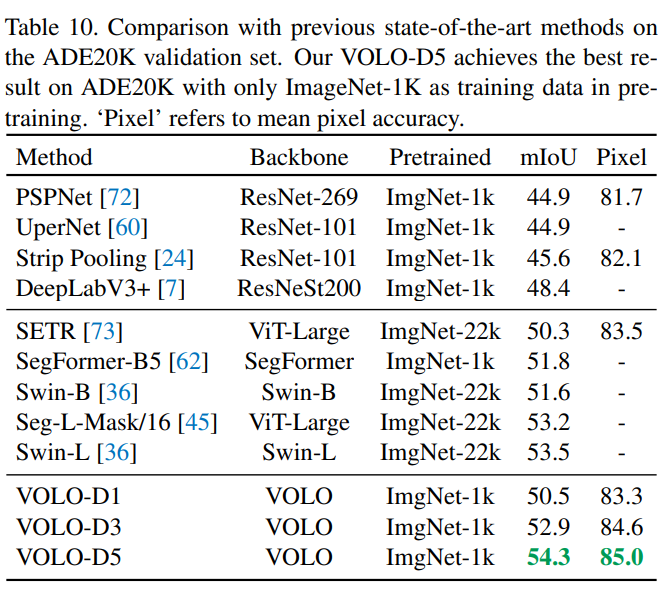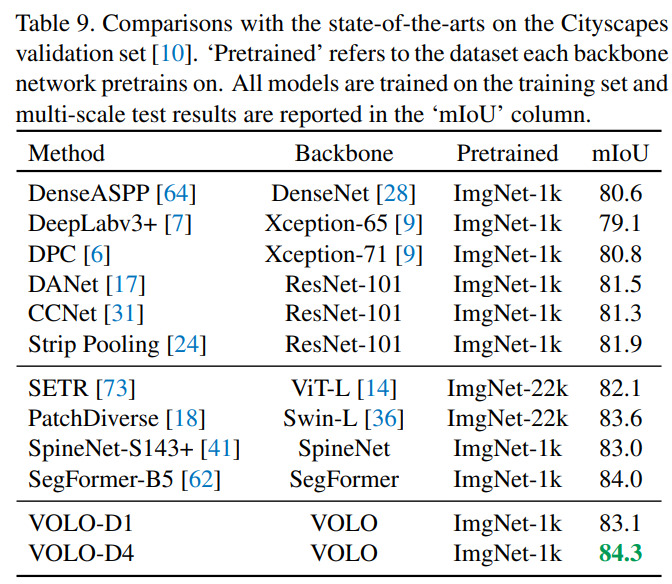# OutLook Attention：具有局部信息感知能力的ViT¶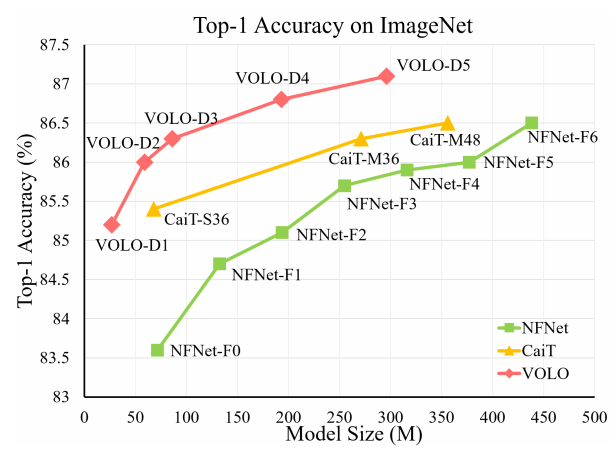# 1. 论文和代码地址¶

VOLO: Vision Outlooker for Visual Recognition

# 2. Motivation¶

“Embedding的图片太大，会导致计算量过大；embedding的图片过小，会导致信息损失太大”，如何在保证合理计算量的情况下，获得更加细粒度的特征表示，这就是本文要解决的问题。

# 3. 方法¶

## 3.1. Outlooker¶

Outlooker的计算步骤如下：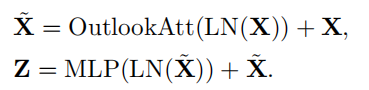## 3.2. Outlook Attention¶

Outlook Attention的核心思想如下：

1）每个空间位置的特征足够丰富，用于产生局部聚合其相邻特征的注意力权重；

2）深层的和局部的空间聚合可以有效地编码细粒度的信息。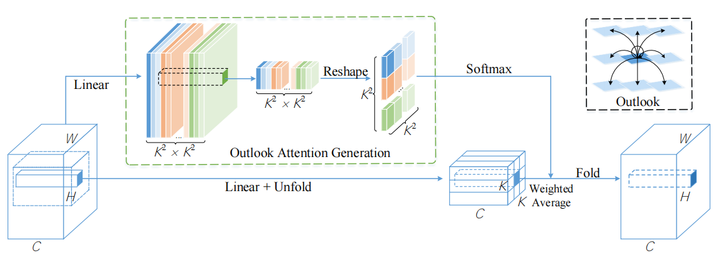### 3.2.1. 生成Attention Map¶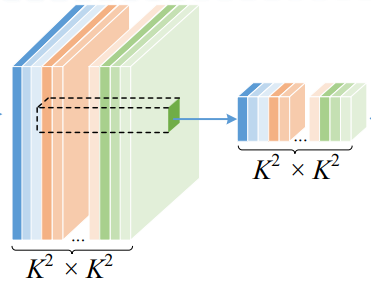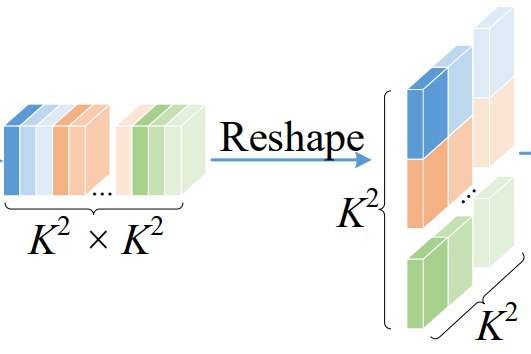### 3.2.2. 映射到新的特征v¶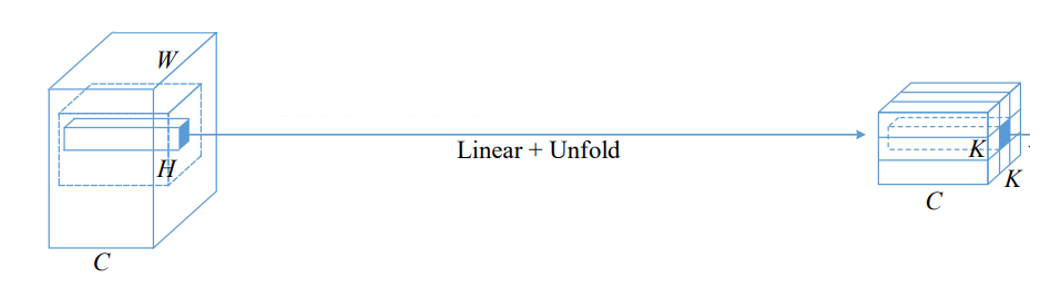### 3.2.3. 获取weighted特征¶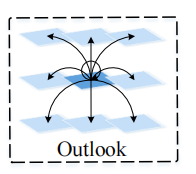Outlook Attention的伪代码如下：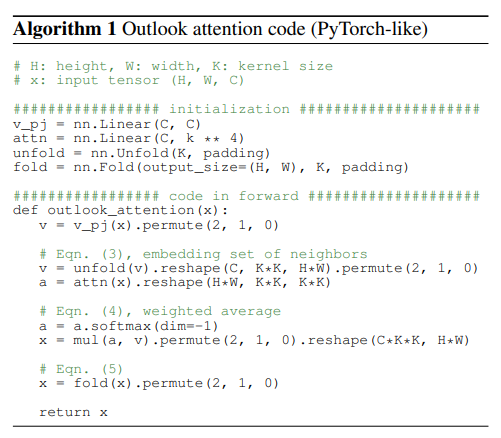pytorch可执行的代码：

class OutlookAttention(nn.Module):

attn_drop=0.1):
super().__init__()
self.dim=dim
self.kernel_size=kernel_size
self.stride=stride

self.v_pj=nn.Linear(dim,dim,bias=qkv_bias)

self.attn_drop=nn.Dropout(attn_drop)
self.proj=nn.Linear(dim,dim)
self.proj_drop=nn.Dropout(attn_drop)

self.pool=nn.AvgPool2d(kernel_size=stride,stride=stride,ceil_mode=True)

def forward(self, x) :
B,H,W,C=x.shape

#映射到新的特征v
v=self.v_pj(x).permute(0,3,1,2) #B,C,H,W
h,w=math.ceil(H/self.stride),math.ceil(W/self.stride)

#生成Attention Map
attn=self.pool(x.permute(0,3,1,2)).permute(0,2,3,1) #B,H,W,C
attn=self.scale*attn
attn=attn.softmax(-1)
attn=self.attn_drop(attn)

#获取weighted特征
out=(attn @ v).permute(0,1,4,3,2).reshape(B,C*self.kernel_size*self.kernel_size,h*w) #B,dimxkxk,H*W
out=F.fold(out,output_size=(H,W),kernel_size=self.kernel_size,
out=self.proj(out.permute(0,2,3,1)) #B,H,W,C
out=self.proj_drop(out)

return out


## 3.3. VOLO的不同变种¶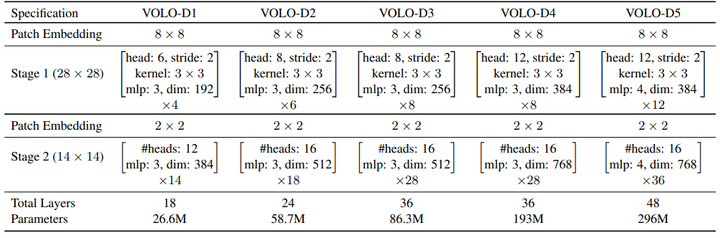## 3.4. 复杂度分析¶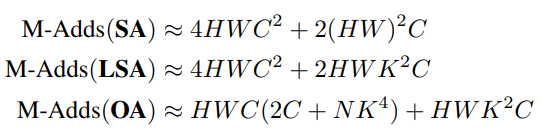# 4. 实验¶